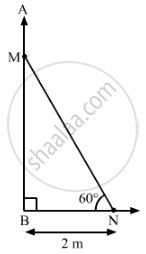# A ladder makes an angle of 60° with the ground when placed against a wall. If the foot of the ladder is 2 m away from the wall, then the length of the ladder (in metres) is: - Mathematics

A ladder makes an angle of 60° with the ground when placed against a wall. If the foot of the ladder is 2 m away from the wall, then the length of the ladder (in metres) is:

(A) 4/sqrt3

(B) 4sqrt3

(C) 2sqrt2

(D)4

#### SolutionIn the figure, MN is the length of the ladder, which is placed against the wall AB and makes an angle of 60° with the ground.

The foot of the ladder is at N, which is 2 m away from the wall.

∴ BN = 2 m

In right-angled triangle MNB:

"Number of favourable outcomes"/"Total number of outcomes"=3/4

Therefore, the length of the ladder is 4 m.
Hence, the correct option is D.

Concept: Heights and Distances
Is there an error in this question or solution?